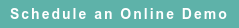# RoyaltyStat Blog

### Posts by Topic

Here, I show that the return on operating assets (ROA) can be specified as the return on investment (ROI).

Economic time series may have one-period autoregressive errors (AR(1)).

Before Newey-West, the Cochrane-Orcutt or the Prais-Winsten AR(1) error correction was pervasive in applied research. Estimating time-dependent economic variables, such as the individual company’s (tested party and comparables) return on operating assets, without the AR(1) error correction will result in inefficient parameter estimates, and the standard errors will be inconsistent. Hence, the unaware reader can begrime the arm’s length range of comparable return on operating assets.

Define P(t) as the operating profit after depreciation (excluding the amortization of intangibles) (OIBAM) of the tested party and the comparables, and K(t) as the year-end property plant & equipment (PPENT ≈ Operating Assets) in audit period t = 1 to T.

A statistical relationship between operating profits and the level of operating assets is expected. Thus, postulate a behavioral operating profit equation subject to random errors:

(1)     P(t) = ρ K(t) + U(t)

where U(t) represents random uncertainty.

Define the (“perpetual inventory”) operating assets growth equation:

(2)     K(t) = β K(t − 1) + G(t)

where G(t) represents the company’s gross investment (CAPX).

The coefficient β = (1 – δ) is one minus the depreciation rate of operating assets.

Lag equation (1) for one period, and obtain:

(3)     P(t − 1) = ρ K(t − 1) + U(t − 1), which implies:

(4)     P(t − 1) − U(t − 1) = ρ K(t − 1)

Substitute (2) into (1), and obtain:

(5)     P(t) = ρ [β K(t − 1) + G(t)] + U(t), or

(6)     P(t) = β ρ K(t − 1) + ρ G(t) + U(t)

Substitute (4) into (6), and obtain:

(7)     P(t) = β [P(t − 1) − U(t − 1)] + ρ G(t) + U(t), or

(8)     P(t) − β P(t − 1) = ρ G(t) + U(t) − β U(t − 1)

Equation (8) is a Koyck regression, which can be simplified by assuming the equilibrium (stability) behavior of operating profits.

Assume that P(t) = P(t − 1), meaning that the level of operating profits is stable between two adjacent periods, and obtain:

(9)     P(t) − β P(t) = ρ G(t) + U(t) − β U(t − 1), or

(10)    P(t) = γ G(t) + V(t)

where the slope of equation (10), that is γ = ρ / (1 – β), is the operating profit multiplier and V(t) is the AR(1) error process.

The AR(1) error corrected return on operating assets of equation (10) is dependent on CAPX, which is the company’s gross investment flow, and not dependent on PPENT (which is a stock variable). Equation (10) means that the return on operating assets equation (1), coupled with the operating assets accumulation equation (2), can become the return on investment.

The gamma (γ) slope multiplier of equation (10) is a pure number (independent of the units in which the flow variables are measured), making cross-company comparisons more reliable.

Suppose that equation (10) is restrictive (because of the assumed operating profit equilibrium). In that case, the reader can move the lagged term β P(t − 1) to the right side of equation (8) and estimate the Koyck regression.

Equation (10) can be estimated using the Cochrane-Orcutt, the Prais-Winsten, or the Newey-West algorithms. The textbook by Carter Hill, William Griffiths, and Guay Lim, Principles of Econometrics (4th edition), Wiley, 2011, covers the major AR(1) error correction algorithms.

The AR(1) error correction algorithms referenced herein are available online in the RoyaltyStat website to ensure the reliable estimation of the individual company-based (tested party and comparables) return on operating assets (flow over stock variables) or the return on investment (more reliable flow over flow variables). The operating profit coefficient γ = ρ / δ of the reduced-form equation (10) is the return on operating assets divided by the depreciation rate of the operating assets employed.

The use of return on operating assets (ROA) is prosaic in transfer pricing. This blog shows that selecting the return on operating assets profit indicator involves sophisticated model specification and not run-of-the-mill econometrics considerations. Misapplied, ROA can be an audit peril in transfer pricing.

Published on Sep 17, 2021 12:19:53 PM

Ednaldo Silva (Ph.D.) is founder and managing director of RoyaltyStat. He helped draft the US transfer pricing regulations and developed the comparable profits method called TNNM by the OECD. He can be contacted at: esilva@royaltystat.com

RoyaltyStat provides premier online databases of royalty rates extracted from unredacted license agreements
and normalized company financials (income statement, balance sheet, cash flow). We provide high-quality data, built-in analytical tools, customer training and attentive technical support.